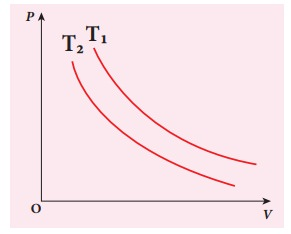Home | | Physics 11th std | Solved Example Problems for Isothermal process

# Solved Example Problems for Isothermal process

Physics : Heat and Thermodynamics - Solved Example Problems for Isothermal process, Solved Example Problems for Thermodynamic Processes

### EXAMPLE 8.16

A 0.5 mole of gas at temperature 300 K expands isothermally from an initial volume of 2 L to 6 L

(a) What is the work done by the gas?

(b) Estimate the heat added to the gas?

(c) What is the final pressure of the gas? (The value of gas constant, R = 8.31 J mol-1 K-1)

### Solution

(a) We know that work done by the gas in an isothermal expansion

Since Âµ = 0.5= 1.369 kJ

Note that W is positive since the work is done by the gas.

(b) From the First law of thermodynamics, in an isothermal process the heat supplied is spent to do work.

Therefore, Q = W = 1.369 kJ. Thus Q is also positive which implies that heat flows in to the system.

(c) For an isothermal process

PiVi = PfVf = ÂµRT=207.75 k Pa

### EXAMPLE 8.17

The following PV curve shows two isothermal processes for two different temperatures and. Identify the higher temperature of these two.### Solution

To determine the curve corresponding to higher temperature, draw a horizontal line parallel to x axis as shown in the figure. This is the constant pressure line. The volumes V1 and V2 belong to same pressure as the vertical lines from V1 and V2 meet the constant pressure line.At constant pressure, higher the volume of the gas, higher will be the temperature. From the figure, as V1 > V2 we conclude T1 > T2. In general the isothermal curve closer to the origin, has lower temperature.

Study Material, Lecturing Notes, Assignment, Reference, Wiki description explanation, brief detail
11th Physics : UNIT 8 : Heat and Thermodynamics : Solved Example Problems for Isothermal process |